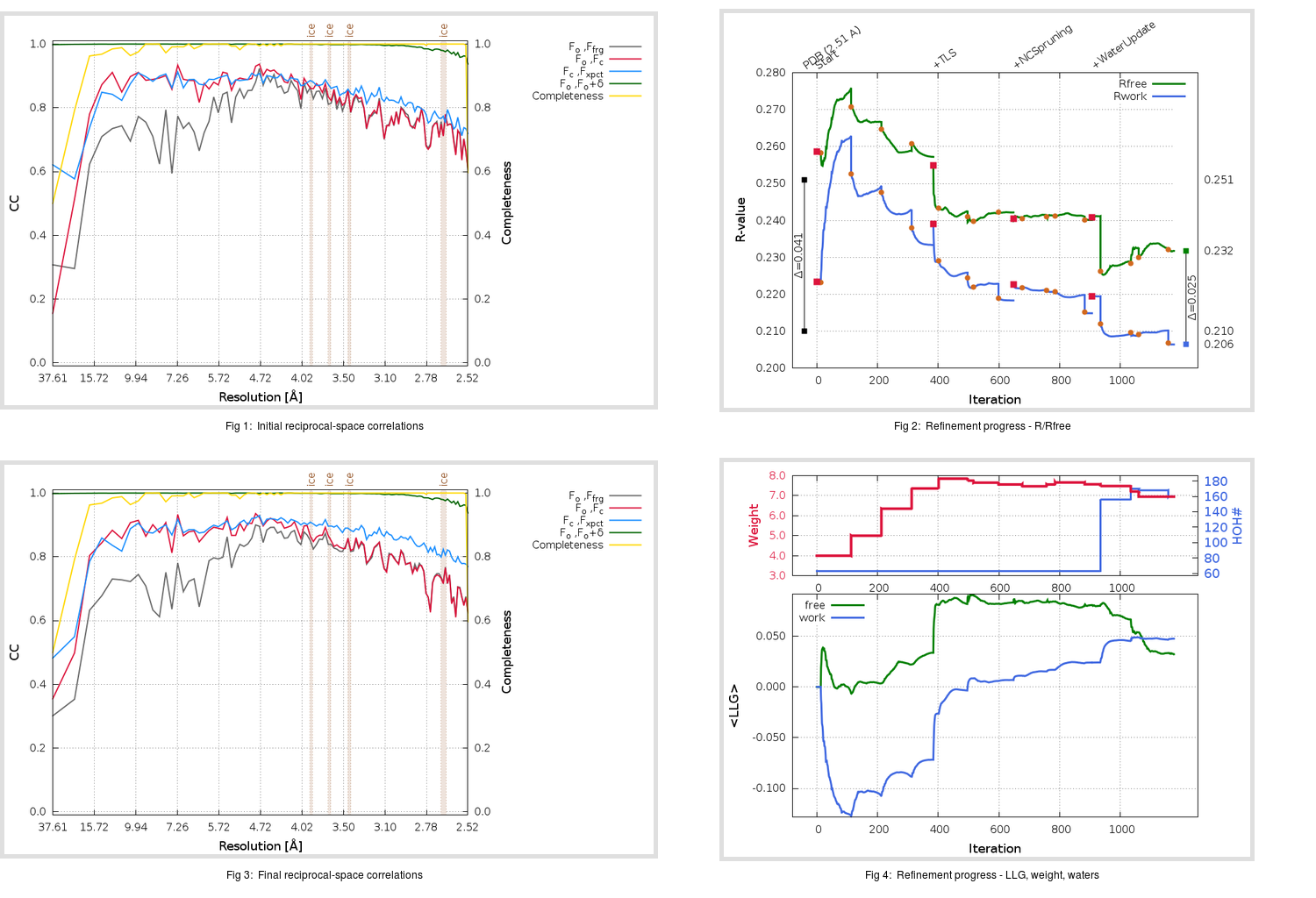Content:

```    Diffraction limits & principal axes of ellipsoid fitted to diffraction cut-off surface:
2.431         1.0000   0.0000   0.0000       a*
2.486         0.0000   1.0000   0.0000       b*
2.476         0.0000   0.0000   1.0000       c*
```

## Deposited

` `
 Date deposited Date data collection Resolution R, Rfree 20130515 20130407 2.51 0.2080 0.2510

Molprobity (CCP4 7.0 version) summary:

```Ramachandran outliers =   0.73 %
favored =  93.69 %
Rotamer outliers      =   8.95 %
C-beta deviations     =     1
Clashscore            =   7.01
RMS(bonds)            =   0.0054
RMS(angles)           =   1.00
MolProbity score      =   2.53
Resolution            =   2.51
R-work                =   0.2080
R-free                =   0.2510
```

```Number of waters      =    63

<B> (all atoms) =   64.34 ( sd =   27.69 ) for       3307 non-hydrogen atoms
<B>   (protein) =   64.42 ( sd =   27.88 ) for       3216 non-hydrogen atoms
<B>     (water) =   49.15 ( sd =    7.36 ) for         63 non-hydrogen atoms
<B>    (others) =   88.43 ( sd =    5.82 ) for         28 non-hydrogen atoms

B min/max       (all non-hydrogen atoms) =   21.80 /  185.21
B min/max   (protein non-hydrogen atoms) =   21.80 /  185.21
B min/max     (water non-hydrogen atoms) =   33.75 /   70.77
B min/max     (other non-hydrogen atoms) =   79.02 /   97.06
```

## BUSTER (re-)refinement

` `

Molprobity (CCP4 7.0 version) summary:

```Ramachandran outliers =   0.97 %
favored =  95.87 %
Rotamer outliers      =   6.58 %
C-beta deviations     =     2
Clashscore            =   2.18
RMS(bonds)            =   0.0109
RMS(angles)           =   1.57
MolProbity score      =   1.90
Resolution            =   2.51
R-work                =   0.2064
R-free                =   0.2317
```

```Number of waters      =   160

<B> (all atoms) =   66.14 ( sd =   23.53 ) for       3404 non-hydrogen atoms
<B>   (protein) =   66.03 ( sd =   23.77 ) for       3216 non-hydrogen atoms
<B>     (water) =   62.11 ( sd =   12.37 ) for        160 non-hydrogen atoms
<B>    (others) =  102.02 ( sd =   13.53 ) for         28 non-hydrogen atoms

B min/max       (all non-hydrogen atoms) =   30.95 /  169.17
B min/max   (protein non-hydrogen atoms) =   30.95 /  169.17
B min/max     (water non-hydrogen atoms) =   33.42 /   97.54
B min/max     (other non-hydrogen atoms) =   86.06 /  116.96
```

Refinement progression:Results:

` `
 File Remark 4KQZ_aB_refine.01_04_refine.pdb.gz exact refinement commands are in header 4KQZ_aB_refine.01_04_refine.mtz.gz including original deposited data and several re-refinement map coefficients 4KQZ_aB_refine.01_04_BUSTER_model.cif.gz including any non-standard compound restraints 4KQZ_aB_refine.01_04_BUSTER_refln.cif.gz